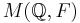# Difference between revisions of "Locally nilpotent not implies normalizer condition"

This article gives the statement and possibly, proof, of a non-implication relation between two group properties. That is, it states that every group satisfying the first group property (i.e., locally nilpotent group) need not satisfy the second group property (i.e., group satisfying normalizer condition)
View a complete list of group property non-implications | View a complete list of group property implications
Get more facts about locally nilpotent group|Get more facts about group satisfying normalizer condition

## Statement

It is possible to have a group$G$ that is a locally nilpotent group (every finitely generated subgroup of it is a nilpotent group) but is not a group satisfying normalizer condition. In other words,$G$ has a proper subgroup$H$ that is self-normalizing:$H = N_G(H)$.

We can construct examples both for$G$ a torsion-free group and for$G$ a p-group for any prime number$p$.

## Proof

### Description of the example

Take a field$F$. Choose$F$ as follows:

• If we want a torsion-free example, take$F = \mathbb{Q}$, or any other field of characteristic zero.
• If we want an example that is a p-group for some prime number$p$, take$F$ to be the field$\mathbb{F}_p$ of$p$ elements, or alternatively, any field of characteristic$p$.

Let$G$ be McLain's group$M(\mathbb{Q}, F)$.

### Proof that it is locally nilpotent$G$ is locally nilpotent because any finitely generated subgroup of$G$ has finite support in the basis$\mathbb{Q}$, i.e., it moves only finitely many basis elements, so it is in a finite-degree unitriangular matrix group over$F$, which is nilpotent.

### Proof that it does not satisfy the normalizer condition

Let$H$ be the subgroup of$G$ generated by the automorphisms:$e_i \mapsto e_i + re_j, r \in F, i < j \le 0$

and:$e_i \mapsto e_i + re_j, r \in F, 0 < i < j$

Basically, we have partitioned the set$\mathbb{Q}$ into two totally ordered subsets: the non-positive and the positive numbers, and are only considering the automorphisms in$G$ that keep each part within itself.

We now need to show that the only automorphisms of the vector space that are in$G$ and preserve$H$ must be within$H$ itself. The basic idea behind the proof (and the key way it differs from the finite case) is that the indexing set is unbounded. In the finite case, the map:$e_{\min} \mapsto e_{\min} + e_{\max}$

would be forced to commute with everything. But in the infinite case, there are no min and max, so any time something from the non-positive side maps to something that has a component on the positive side, we can find things below the non-positive number and above the positive number to provide a contradiction.